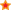for Secondary Education in the UK.Find Resources By Subjects
Information

# HBR Maths

Price: £0.00
Age Range: 11-16 and ABE

A huge range of Maths assessments from prolific author Helen Barnes. They become more and more popular as time goes on.

These assessments are run through Yacapaca, which may charge a subscription fee based on your overall level of usage

## Order this publication:

Quantity Format
Choose delivery option (Yacapaca) at checkout.
Author(s): Helen Barnes
Code: HBRM
Popularity rank: None
Average Customer Review:based on 1 review

# Contents List

• Algebra simplifying and brackets
• Angle Properties
• Angles on a straight line and in a triangle
• Angles, 2nd february 2007
• Angles, Parallel lines and similarity
• Area
• Area and Perimeter
• Area and Perimeter of Rectangles
• Area of triangles and parallelograms
• Averages 2
• Averages, 16th march 2007
• BIDMAS
• BIDMAS and Substitution
• Conversions and units
• Decimals, Factors and Multiples
• Expanding brackets
• Factors and Multiples
• Fractions
• Fractions and Percentages of a Number
• Fractions revision, 2nd February 2007
• Fractions, Decimals and Percentages
• Functions and mappings
• Homework, 26th January, Angles
• Indices and Standard Form
• Inequalities
• Inequalities 1
• Interior and Exterior angles
• Katie's test
• Learning Survey
• Level 4-6 revision 2
• LUCY and EMMA
• Mean, Median, Mode and Range
• mental Test A 2006
• Multiply and Divide by 10, 100 and 1000
• Negative Numbers
• Negative Numbers 9th February 2007
• Negative Numbers, Four Operations and Ordering
• nth term of a sequence
• Nth term of a sequence, linear
• Nth term of a sequence, Year 7
• Number 1 Odd and Even
• Our first online homework
• Our First Online Test
• Parallel lines and similarity
• Pie Charts
• Probability
• Product of Prime Factors
• Proportion and construction
• Ratio and BIDMAS
• Ratio and Proportion
• Ratio, 19th April 2007
• Ratio, simplifying and dividing
• Reflections and rotations
• Revision. 9th February 2007
• Rounding and Estimation
• Rounding and Factors
• Rounding, whole numbers and decimal places
• Sequences
• Sequences homework, 26th January
• Sequences, generating and describing
• Sequences, Nth terms and generating
• Simplifying and Equivalent fractions
• Solving Equations
• Solving Equations 2
• Solving Equations A,B and C
• Solving Equations and Substitution, 1
• Solving Equations Including Negatives
• Speed and Conversions
• Speed Distance Time
• Standard Form 1
• Substitution and Solving Equations
• survey

## Reviews

01/10/08

### Aidan McCanny at SELB

The HBR course provides an excellent resource for Maths teachers. The variety of question types along with diversity of topics makes this very useful.